# 基于HMM的语音识别(一)

Posted by lili on

HMM模型自从1980年代被用于语音识别以来，一直都是实际语音识别系统的主流方法。即使到了今天，End-to-End的语音识别系统不断出现，在工业界很多主流的系统仍然还是在使用基于HMM模型的方法，当然很多情况引入了深度神经网络用来替代传统的GMM模型。因此我们首先来了解一些经典的基于HMM模型的语音识别系统。

## 语音产生过程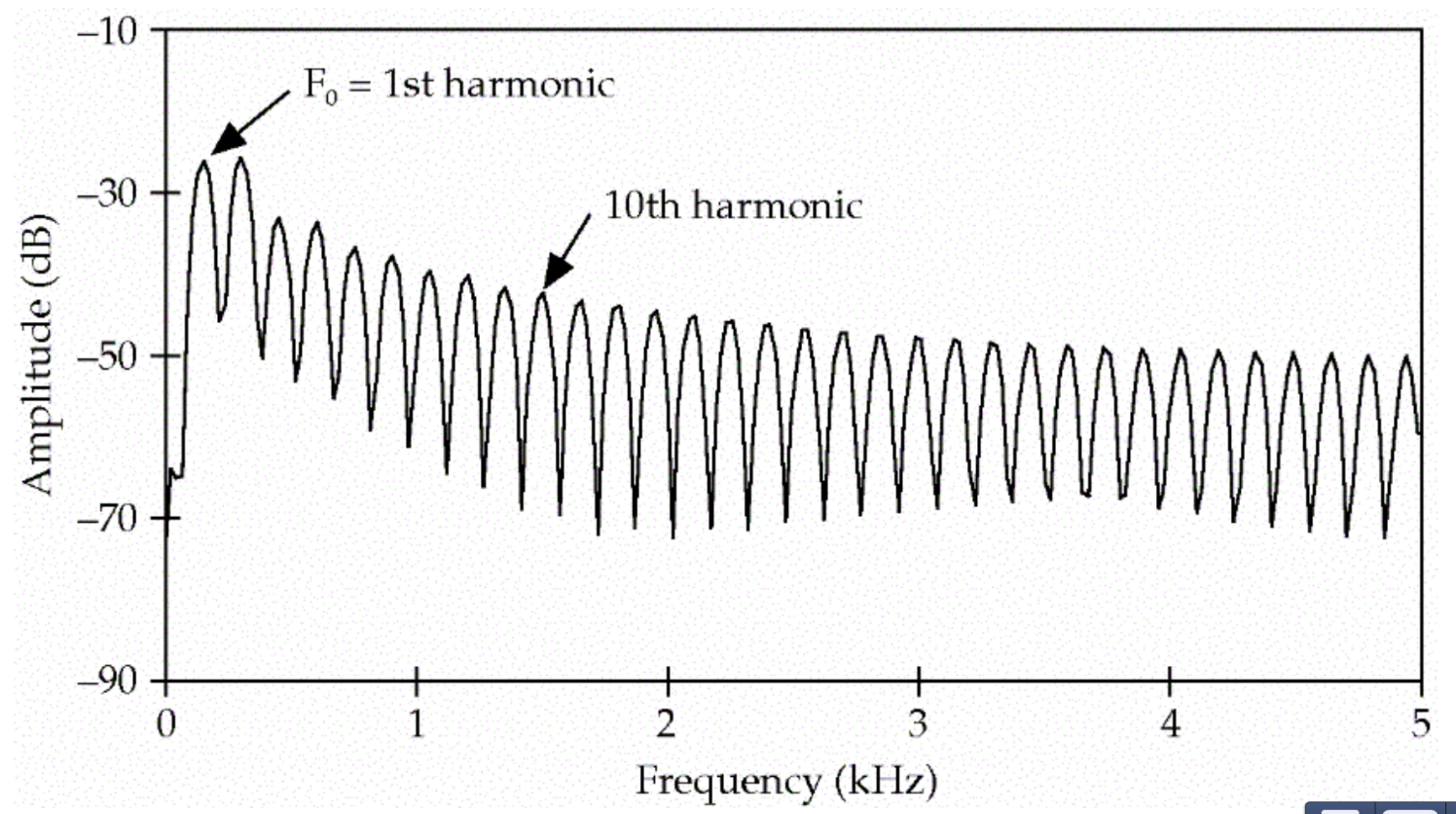图：Harmonics 图片来自课程cs224图：source-filter模型 图片来自课程cs224

## HMM模型简介

HMM模型的目的就是根据观察的序列，估计“最可能”的模型(就是这两个概率)。那什么叫最可能的模型呢？这两个概率都有无穷(或者只是很多)种可能，假设Jason吃雪糕的数量只能是$O={1,2,3}$，$p(O=1|H),p(O=2|H),p(O=3|H)$表示热天吃一、二和三支雪糕的概率，那么可能的一种概率分布是(0.1,0.2,0.7)，也可能是(0.1,0.3,0.6)，…，理论上有无穷种可能。但是模型一旦确定，我们可以计算在这个模型下看到某个序列的概率(计算方法后面会介绍)。存在一个(或者多个)模型使得这个概率最大，那么我们就可以认为这个模型是“最优”的。理论上我们可以“遍历”所有可能的模型，然后找到“最优”的模型，但实际上可能的模型参数是无穷的，因此我们需要更聪明的办法来找到这个最优的模型。下面我们先形式化的定义HMM以及HMM模型的三个问题。

### 定义

HMM包括如下部分：

• $Q=\{Q_1,Q_2,…,Q_N\}$

包含N个状态的状态集合Q

• $A=\{a_{11},a_{12},…,a_{1N},…,a_{N1},a_{N2},…,a_{NN}\}$

状态转移概率矩阵，要求$\sum_{j=1}^Na_{ij}=1$

• $O=o_1o_2…o_T$

观察序列，也就是前面提取的特征，为了简单，我们这里先假设观察是离散(有限)的。这里每个$o_t$属于字典集合$\mathcal{V}=\{v_1,v_2,…,v_V\}$。

• $B=b_i(o_t)$

观察似然(observation likelihoods)，也叫发射概率(emission probabilities)，表示在状态i看到观察$o_t$的概率

• $q_0, q_F$

初始和结束状态，同时还包括初始状态到N个状态的跳转概率$q_{01},..,q_{0N}$和N个状态到结束状态的跳转概率$q_{1F},..,q_{NF}$，注意其它状态是不能跳到初始状态的，结束状态也不能跳到其它状态。

$P(q_i|q_1,...,q_{i-1})=P(q_i|q_{i-1})$

$P(o_i|q_1,...,q_T,o_1,...,o_T)=P(o_i|q_i)$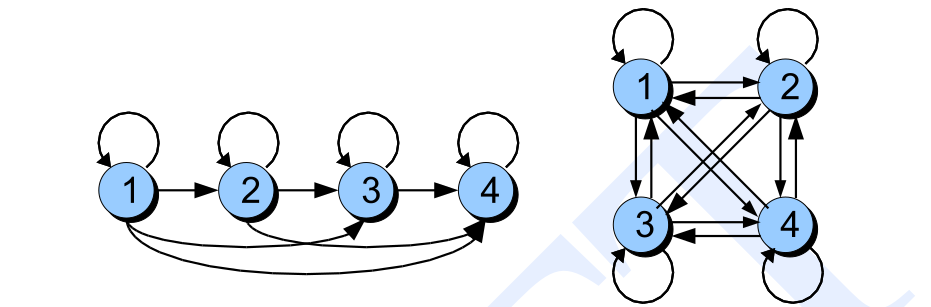图：Bakis和各态历经的HMM

HMM有三个基本问题：

• 似然(Likelihood) 给定HMM$\lambda=(A,B)$和观察序列O，计算似然$P(O |\lambda)$
• 解码(Decoding) 给定HMM$\lambda=(A,B)$和观察序列O，寻找最优的状态序列Q
• 学习(Learning) 给定观察序列，寻找最优的模型参数$\lambda=(A,B)$

### 似然：前向算法(forward algorithm)

$P(3,1,3|hot,hot,cold)=P(3|hot)P(1|hot)P(3|cold)$

$P(3|hot)P(1|hot)P(3|cold)=0.4*0.2*0.1=0.008$

$\begin{split} P(O,Q) & =P(O|Q)P(Q) \\ & = P(q_0,q_1,q_2,...,q_T,q_F) P(o_1,o_2,...,o_T|q_0,q_1,q_2,...,q_T,q_F) \\ & = P(q_0) \prod_{i=1}^{T}P(q_i|q_0,...q_{i-1}) \prod_{i=1}^{T}P(o_i|q_0,...,q_F,o_1,...,o_{i-1}) \\ & = 1 \times \prod_{i=1}^{T}P(q_i|q_{i-1}) P(q_F|q_T) \prod_{i=1}^{T}P(o_i|q_i) \end{split}$

$P(O)=\sum_QP(O,Q)$

$\begin{split} q_1,q_2,q_1 \\ q_1,q_2,q_2 \\ q_1,q_2,q_3 \end{split}$

$\begin{split} P(o_1,o_2,o_3|q_1,q_2,q_1) =P(q_1|q_0)P(q_2|q_1)P(q_1|q_2)P(q_F|q_1) P(o_1|q_1)P(o_2|q_2)P(o_3|q_1) \\ P(o_1,o_2,o_3|q_1,q_2,q_2) =P(q_1|q_0)P(q_2|q_1)P(q_2|q_2)P(q_F|q_2) P(o_1|q_1)P(o_2|q_2)P(o_3|q_2) \\ P(o_1,o_2,o_3|q_1,q_2,q_3) =P(q_1|q_0)P(q_2|q_1)P(q_3|q_2)P(q_F|q_3) P(o_1|q_1)P(o_2|q_2)P(o_3|q_3) \end{split}$

$\alpha_t(j)=P(o_1,o_2,...,o_t,q_t=j|\lambda)$

$\alpha_t(j)=\sum_{i=1}^{N}\alpha_{t-1}(i)a_{ij}b_j(o_t)$

t-1时刻处于状态1并且观察到$o_1,…,o_{t-1}$的概率就是$\alpha_{t-1}(1)$，而t-1时刻处于状态2并且观察到$o_1,…,o_{t-1}$的概率就是$\alpha_{t-1}(2)$，…。因此：

$\alpha_t(j)=\alpha_{t-1}(1)a_{1j}b_j(o_t) + ...+ \alpha_{t-1}(N)a_{Nj}b_j(o_t)=\sum_{i=1}^{N}\alpha_{t-1}(i)a_{ij}b_j(o_t)$

$\alpha_1(j)=a_{0j}b_j(o_1), 1 \le j \le N$

$P(O|\lambda)=\alpha_T(q_F)=\sum_{i=1}^{N}\alpha_T(i)a_{iF}$

Forward Trellis展示了前向算法的计算过程，比如预测天气的例子，如下图所示。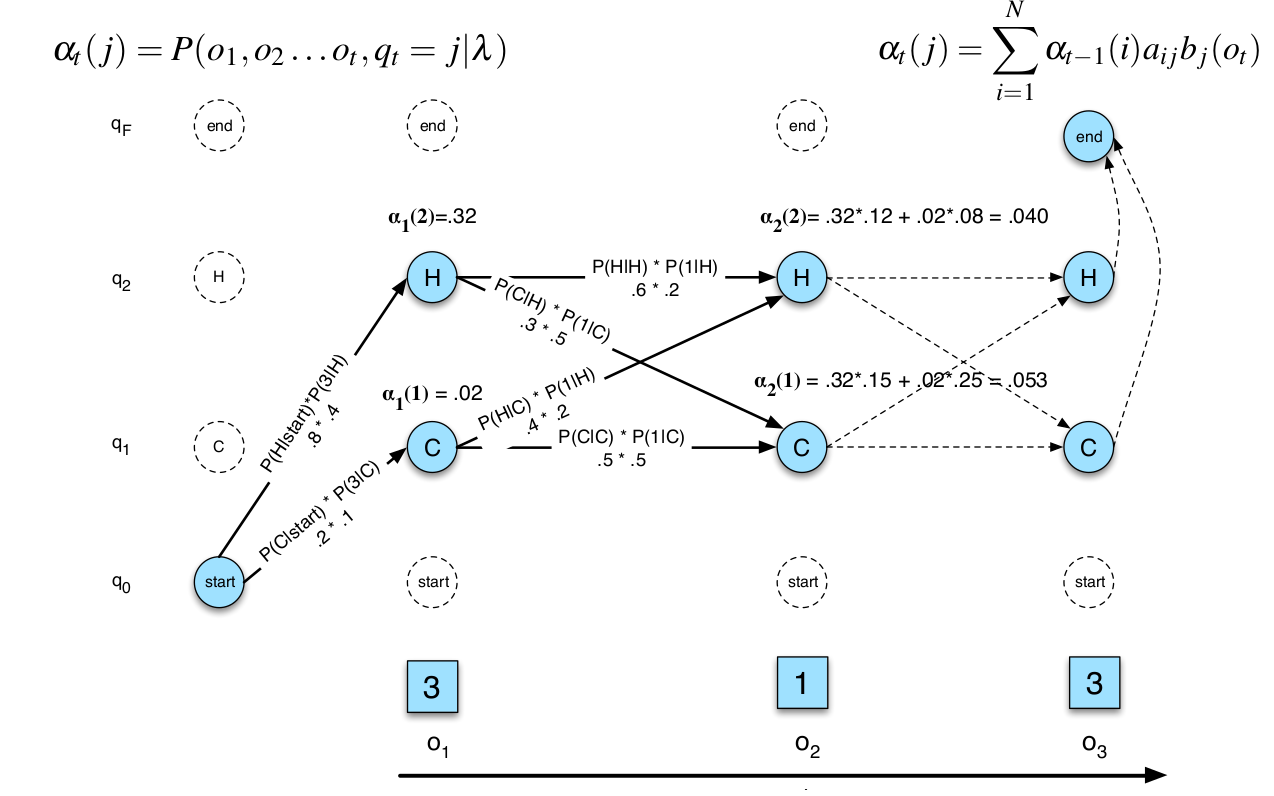图：Forward Trellis

### 解码：Viterbi算法

$v_t(j)=\underset{q_1...q_{t-1}}{max}P(q_0,q_1,...,q_{t-1},o_1,...,o_t, q_t=j|\lambda)$

$P(q_0,q_1,…,q_{t-1},o_1,…,o_t, q_t=j|\lambda)$是观察为$o_1,…,o_t$，状态为$q_0,…,q_{t-1},j$的联合概率。$v_t(j)$它要求在所有长度为t的路径中寻找最大的联合概率(当然也需要找到这条路径)，要求是t时刻的状态是一定为j。

$v_t(j)=max_{i=1}^N v_{t-1}(i)a_{ij}b_j(o_t)$

$\begin{split} v_1(j) & =a_{0j}b_j(o_1),\text{其中} 1 \le j \le N \\ bt_1(j) &=0 \end{split}$

$\begin{split} v_t(j)=max_{i=1}^N v_{t-1}(i)a_{ij}b_j(o_t), \text{其中} 1 \le j \le N , 1 \le t \le T \\ bt_t(j)=argmax_{i=1}^N v_{t-1}(i)a_{ij}b_j(o_t), \text{其中} 1 \le j \le N , 1 \le t \le T \end{split}$

$\begin{split} \text{最优路径的概率：} P*=v_T(q_F)=max_{i=1}^Nv_T(i)a_{iF} \\ \text{最优路径在T时刻的状态：} q_T*=bt_T(q_F)=argmax_{i=1}^Nv_T(i)a_{iF} \end{split}$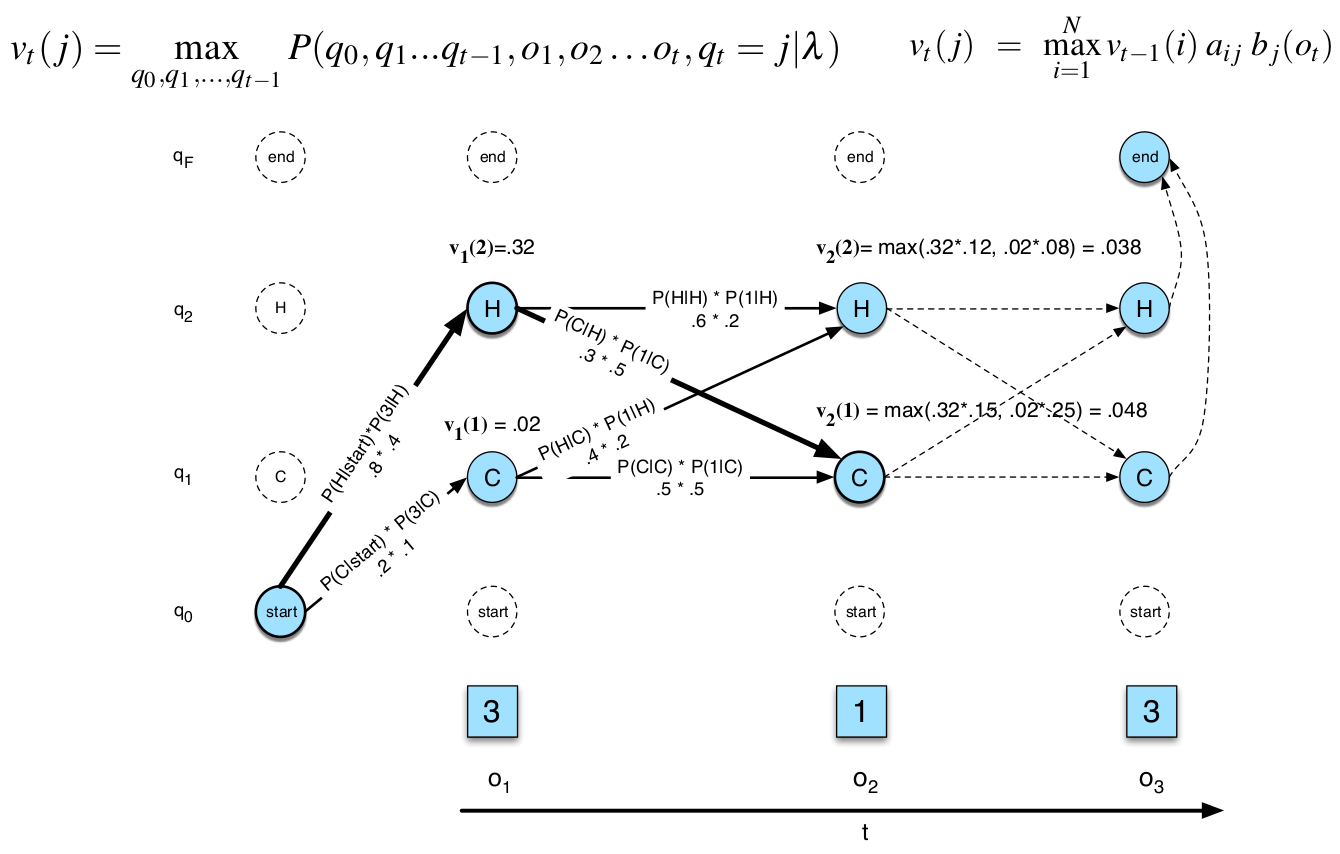图：Viterbi Trellis

### 学习：前向后向(forward-backward)算法

$\hat{a}_{ij}=\frac{C(i \rightarrow j)}{\sum_{q \in Q} C(i \rightarrow q)}$

$\hat{b}_j(v_k)=\frac{\sum_{t=1}^T 1_{[o_t=v_k and s_t=j]} }{\sum_{t=1}^T 1_{[s_t=j]} }$

Viterbi训练算法的问题在于它会为每一个时刻找一个固定的“最优”的状态，但很多时候某个观察到底是属于哪个状态是很难确定的。尤其在迭代的早期，本来模型就不好，用它来找到的“最优”状态序列就不准，然后用不准的状态来估计模型参数就更不准了。所以更好的办法是用当前的模型计算每个时刻的状态的概率分布，这样可以避免前面的问题。那怎么计算每个隐状态的概率分布呢？这就需要用到前向后向算法了。

$\label{eq:fb-gamma} \gamma_t(j)=P(q_t=j|O,\lambda)$

$\hat{b}_j(v_k)=\frac{\sum_{t=1}^T 1_{[o_t=v_k]} \gamma_t(j) }{\sum_{t=1}^T \gamma_t(j)}$

$\epsilon_t(i,j)=P(q_t=i,q_{t+1}=j|O,\lambda)$

$\begin{split} \hat{a_{ij}} & =\frac{\sum_{t=1}^{T-1} \epsilon_t(i,j) }{\sum_{t=1}^{T-1}\sum_{k=1}^{N}\epsilon_t(i,k)} \\ & =\frac{\sum_{t=1}^{T-1} \epsilon_t(i,j) } {\sum_{t=1}^T \gamma_t(i)} \end{split}$

$\gamma_t(i)=\sum_{k=1}^{N}\epsilon_t(i,k)$

$\beta_t(i)=P(o_{t+1}, ..., o_T|q_t=i,\lambda)$

$\beta_T(i)=a_{iF}, 1 \le i \le N$

$\beta_t(i)=\sum_{j=1}^{N}a_{ij}b_j(o_{t+1})\beta_{t+1}(j), 1 \le i \le N, 1 \le t < T$

$P(O|\lambda)=\beta_1(0)=\alpha_T(q_F)=\sum_{j=1}^{N}a_{0j}b_j(o_1)\beta_1(j)$

$\begin{split} \gamma_t(j) & =P(q_t=j|O,\lambda) \\ & = \frac{P(q_t=j,O|\lambda)}{P(O|\lambda)} \\ & = \frac{P(o_1,...,o_t,o_{t+1},...,o_T, q_t=j|\lambda)}{P(O|\lambda)} \\ & = \frac{P(o_1,...,o_t,q_t=j|\lambda) P(o_{t+1},...,o_T|q_t=j,\lambda)}{P(O|\lambda)} \\ & = \frac{\alpha_t(j)\beta_t(j)}{P(O|\lambda)} \end{split}$

$\begin{split} P(X,Y|Z) & =P(X|Y,Z)P(Y|Z) \\ P(X|Y,Z) & =\frac{P(X,Y|Z)}{P(Y|Z)} \end{split}$

$\begin{split} P(X,Y) & =P(X|Y)P(Y) \\ P(X|Y) & =\frac{P(X,Y)}{P(Y)} \end{split}$

$\begin{split} \epsilon_t(i,j) & = P(q_t=i, q_{t+1}=j|O,\lambda) \\ & = \frac{P(q_t=i, q_{t+1}=j, O|\lambda)}{P(O|\lambda)} \\ & = \frac{P(q_t=i, q_{t+1}=j, o_1,...,o_t,o_{t+1},..,o_T|\lambda)}{P(O|\lambda)} \\ & = \frac{P(o_1,...,o_t, q_t=i, q_{t+1}=j, o_{t+1},..,o_T|\lambda)}{P(O|\lambda)} \\ & = \frac{P(o_1,...,o_t, q_t=i|\lambda)P(q_{t+1}=j, o_{t+1},..,o_T|o_1,...,o_t, q_t=i,\lambda)}{P(O|\lambda)} \\ & = \frac{\alpha_t(i)}{P(O|\lambda)} P(q_{t+1}=j, o_{t+1},..,o_T|o_1,...,o_t, q_t=i,\lambda) \\ & = \frac{\alpha_t(i)}{P(O|\lambda)} P(o_{t+1},..,o_T|q_{t+1}=j, o_1,...,o_t, q_t=i,\lambda)P(q_{t+1}=j|o_1,...,o_t, q_t=i,\lambda) \\ & = \frac{\alpha_t(i)}{P(O|\lambda)} P(o_{t+1},..,o_T|q_{t+1}=j,\lambda)P(q_{t+1}=j|q_t=i,\lambda) \\ & = \frac{\alpha_t(i)}{P(O|\lambda)} P(o_{t+1}|q_{t+1}=j,\lambda)P(o_{t+2},...,o_T|q_{t+1}=j,\lambda) a_{ij} \\ & = \frac{\alpha_t(i)}{P(O|\lambda)}a_{ij}b_j(o_{t+1})\beta_{t+1}(j) \\ & = \frac{\alpha_t(i)a_{ij}b_j(o_{t+1})\beta_{t+1}(j)}{P(O|\lambda)} \end{split}$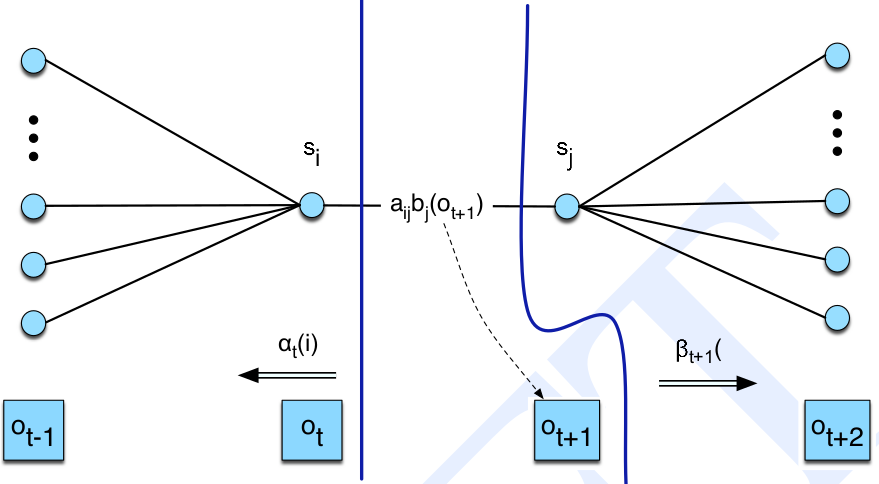图：计算$\epsilon$的图示